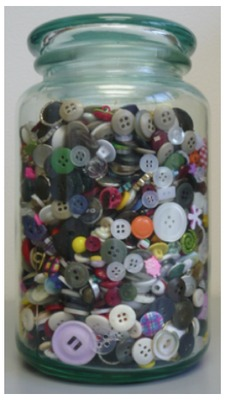# Button Diameters

Alignments to Content Standards: 4.MD.B.41. With a partner or group, gather a handful of round buttons from a diverse collection, and use a ruler to measure the diameter of each button to the nearest eighth-inch.
2. Make a line plot of button diameters, marking your scale in eighth-inch increments.
3. What is the most common diameter in your collection? How does that compare with the collection from another group?
4. Now measure the diameters of these same buttons to the nearest quarter-inch.
5. Make a line plot of button diameters, marking your scale in quarter-inch increments.
6. Describe the differences between the two line plots you created. Which one gives you more information? Which one is easier to read?

## IM Commentary

The purpose of this task is for students to measure lengths to the nearest eighth and quarter-inch and to record that information in a line plot. Both 4th graders and 5th graders use line plots to record measurement data given in fractions of a unit. Both 4.MD.4 and 5.MD.2 include adding and subtracting values given in the line plot. For 4th grade, this includes adding and subtracting fractions with like denominators. For 5th grade, this includes any operations with fractions through division of a whole numbers by a unit fraction (or vice versa; see 5.NF.A and 5.N.B).

The teacher should have a large assortment of round buttons for this activity and divide the students into partners or groups of three or four students. Each group should use 20 to 30 buttons for this activity.

This task does not explicitly ask operations-based questions, but it would be a strong task to introduce line plots with fractions. The teacher might consider prompting students to find the difference between the diameter of the biggest button and smallest button or to find the total length of the 5 biggest buttons placed right next to each other to address the other aspects of the standard.

## Solution

1. Here are the measurements from a sample of 25 buttons that I collected:

 Diameter to nearest eighth-inch Count $\frac38$ $\frac12$ $\frac58$ $\frac34$ $1 \frac18$ 7 6 6 5 1
2. Below is a line plot based on the data collected in part a.3. In this collection, the most common diameter is $\frac38$ of an inch. That may vary some from other groups.

4. Here are the measurements from the same sample of 25 buttons from part a.

 Diameter to nearest quarter-inch Count $\frac14$ $\frac12$ $\frac34$ $1$ 1 18 5 1
5. Below is a line plot based on the data in part d.6. From the line plots shown above, the second shows less variation among the diameters, and clearly shows that the most common diameter, to the nearest quarter-inch, is $\frac12$ inch. The first line plot gives more information about the diameters, and shows a cluster of buttons with diameters that round to $\frac38$ inch to $\frac34$ inch.